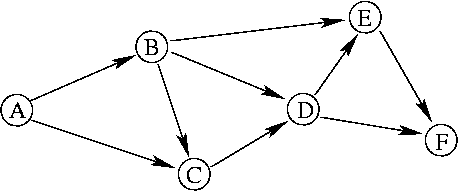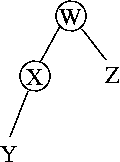## COS 402: Introduction to Artificial Intelligence

### Homework #1 Turing Test and Search

#### Fall 2004 Due: Wednesday, September 22

This assignment consists of written exercises only (the next assignment will also involve programming).  All problems are worth 10 points, except problem 3 which is worth 15 points.  See the assignments page for information on when and where to submit written exercises.

Remember that if you do not already know Java, you should be learning it at this time (for instance, by reading Java in a Nutshell).

1.  There are many "chatterbots" on the web that attempt to carry on human-level conversations, often within a limited domain.  These include:

Have a "conversation" with one or more of these chatterbots.  Try to test what they can or cannot do, what they are good at, and what they are not so good at.  See what you can learn about how they work.  Then briefly (say, in three paragraphs or less) describe and analyze your experience.

2.  Problem 3.7 in R&N (where successor function refers simply to the actions that are possible from each state).  Do any two of the four parts of this problem.

3.  Consider the following search problem:The states are: A, B, C, D, E and F.  The initial state is A.  The goal state is E.  The actions permit movement along directed edges (for instance, from B, we can move to C, D or E).  Assume unit step cost.

Draw the search tree that would be produced by running each of the search algorithms BFS, DFS and IDS on this problem.  When multiple nodes are added all at once to the fringe (or queue), assume they are added in such a way as to be removed later from the fringe in alphabetical order.  If a goal state is reached, indicate the solution (sequence of states) that was found and whether or not it is optimal.  For instance, the answer (for a different graph) might look like this:solution: W Z (optimal)

For IDS, give separate answers for each choice of the depth parameter.